The CATMOD Procedure
 Background: The Underlying Model

The CATMOD procedure analyzes data that can be represented by a two-dimensional contingency table. The rows of the table correspond to populations (or samples) formed on the basis of one or more independent variables. The columns of the table correspond to observed responses formed on the basis of one or more dependent variables. The frequency in the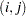th cell is the number of subjects in theth population that have theth response. The frequencies in the table are assumed to follow a product multinomial distribution, corresponding to a sampling design in which a simple random sample is taken for each population. The contingency table can be represented as shown in Table 28.1.

Table 28.1 Contingency Table Representation

Response

Sample

1

2Total

1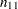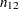2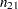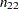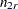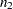s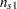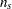For each sample, the probability of theth response () is estimated by the sample proportion,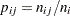. The vector () of all such proportions is then transformed into a vector of functions, denoted by. Ifdenotes the vector of true probabilities for the entire table, then the functions of the true probabilities, denoted by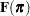, are assumed to follow a linear modelwhere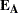denotes asymptotic expectation,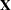is the design matrix containing fixed constants, andis a vector of parameters to be estimated.

PROC CATMOD provides two estimation methods:

• The weighted least squares method minimizes the weighted residual sum of squares for the model. The weights are contained in the inverse covariance matrix of the functions. According to central limit theory, if the sample sizes within populations are sufficiently large, the elements of
and(the estimate of) are distributed approximately as multivariate normal. This allows the computation of statistics for testing the goodness of fit of the model and the significance of other sources of variation. For details of the theory, see Grizzle, Starmer, and Koch (1969) or Koch et al. (1977, Appendix 1). Weighted least squares estimation is available for all types of response functions.

• The maximum likelihood method estimates the parameters of the linear model so as to maximize the value of the joint multinomial likelihood function of the responses. Maximum likelihood estimation is available only for the standard response functions, logits and generalized logits, which are used for logistic regression analysis and log-linear model analysis. Two methods of maximization are available: Newton-Raphson and iterative proportional fitting. For details of the theory, see Bishop, Fienberg, and Holland (1975).

Following parameter estimation, hypotheses about linear combinations of the parameters can be tested. For that purpose, PROC CATMOD computes generalized Wald (1943) statistics, which are approximately chi-square distributed if the sample sizes are sufficiently large and the null hypotheses are true.Previous Page | Next Page | Top of Page